### Home > MC2 > Chapter 5 > Lesson 5.2.2 > Problem5-56

5-56.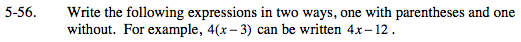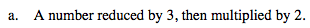Reducing by 3 means you first need to subtract 3 from a number. We will call this unknown number x. Then you will multiply that value by 2.

A number reduced by 3: (x − 3)

Then multiplied by 2: 2(x − 3)

Use the Distributive Property to multiply every term inside the parentheses by 2.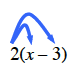2x − 6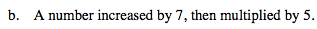See part (a).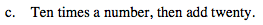Ten times a number is 10x. Then add twenty to that to get: 10x + 20

Since 10 is a factor of both terms, we can factor out a 10 to get the following expression: 10(x + 2)